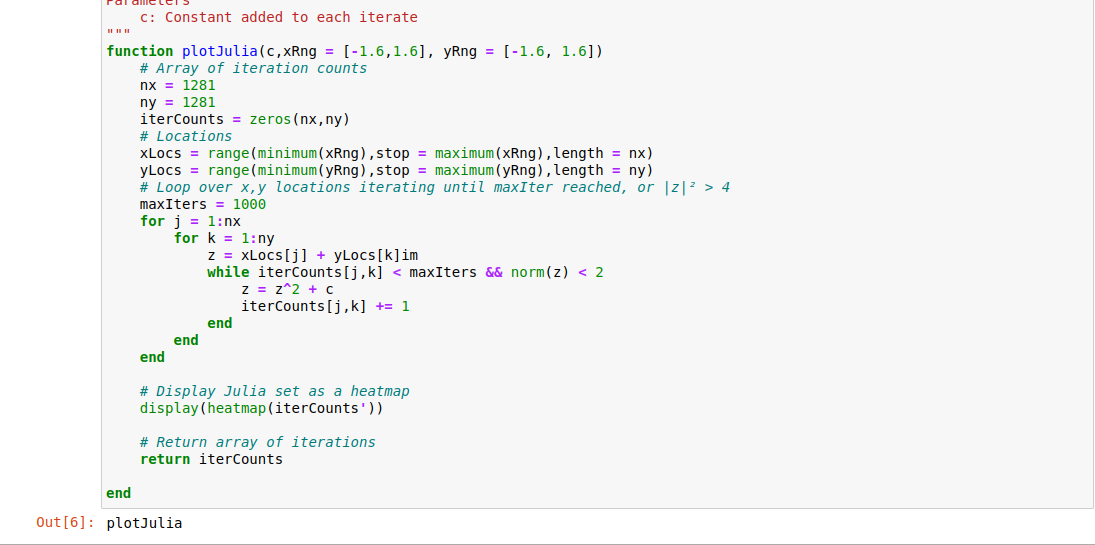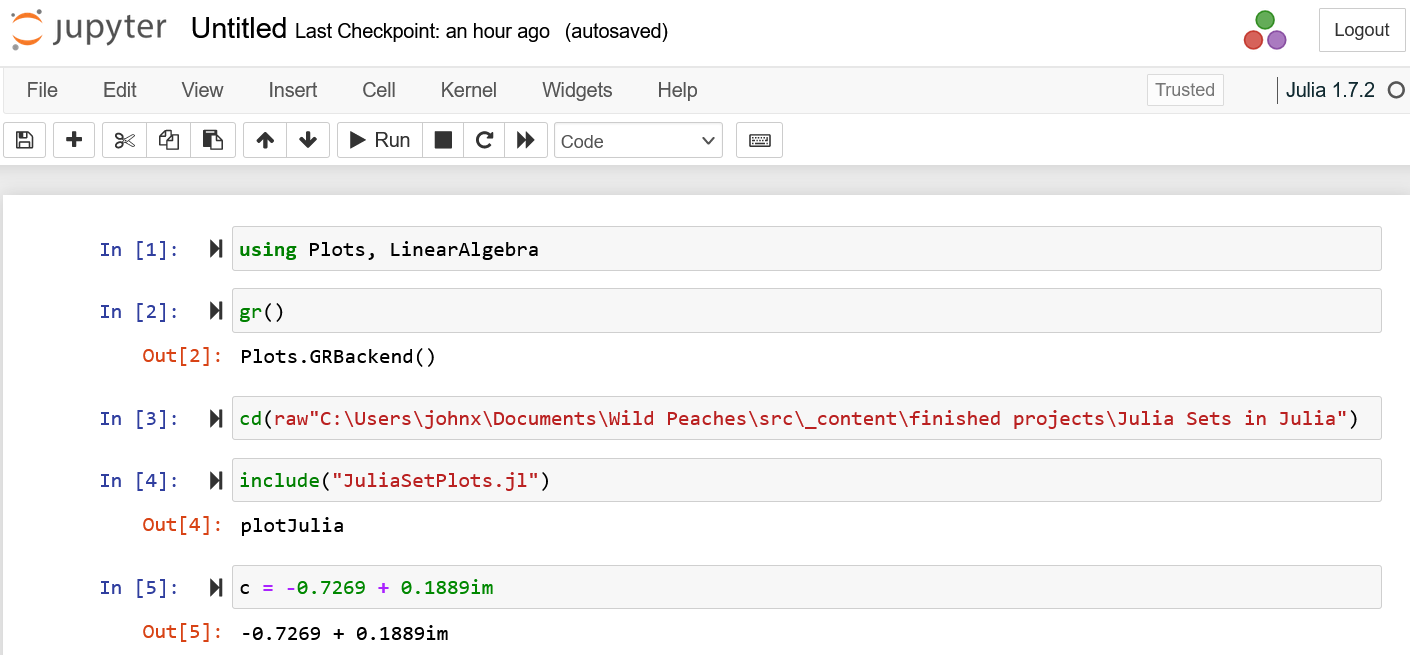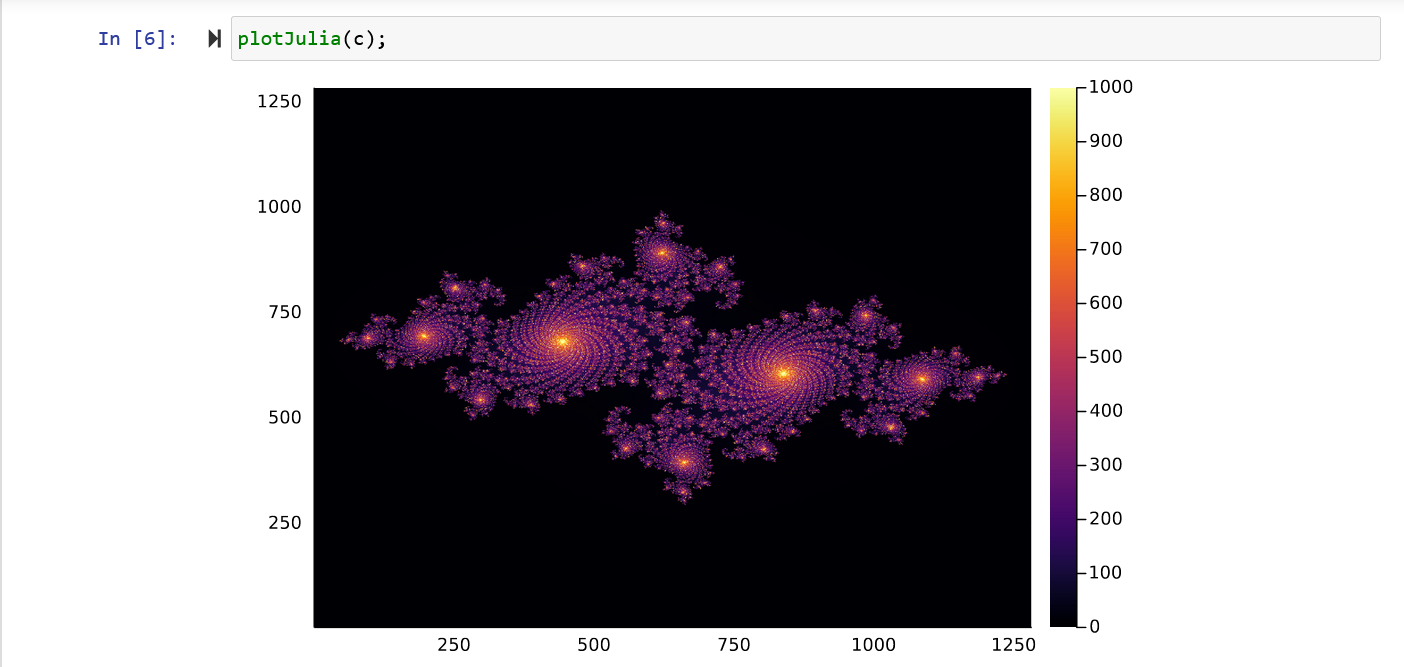{{ message }}

Instantly share code, notes, and snippets.

# XerxesZorgon/JuliaSetPlots.jl

Created Feb 25, 2022
Plots trajectories and filled Julia sets
This file contains bidirectional Unicode text that may be interpreted or compiled differently than what appears below. To review, open the file in an editor that reveals hidden Unicode characters. Learn more about bidirectional Unicode characters
 #= Plot Julia functions Load with: include("JuliaSetPlots.jl") Written by: John Peach 13-Feb-2022 Wild Peaches Julia sets are defined as z_{n+1} = zₙ² + c where zₙ ∈ ℂ and c = x₀ + iy₀ Mandelbrot sets are similar, but z₀ is fixed, and c ∈ ℂ. =# # Required packages using Plots, LinearAlgebra gr() """ plotIterates Plots iteratations of the Julia function from in initial point z₀ ∈ ℂ and parameter c Parameters z₀: Starting point c: Control parameter n: Number of iteratations Returns Plot of n iteratations """ function plotIterates(z₀,c,n) # Initialize trajectory zₙ = length(z₀) traj = zeros(ComplexF64,n,zₙ) traj[1,:] .= z₀ # Generate trajectory for k = 1:zₙ for j = 2:n traj[j,k] = traj[j-1,k]^2 + c end end # Plot trajectory. The display function is needed inside a function. crvLabel = Array{String}(undef,zₙ) for k = 1:zₙ crvLabel[k] = string("z₀ = ", z₀[k]) end # Note permation of label string. # Ref: https://discourse.julialang.org/t/transpose-of-an-array-of-strings/40431/8 crvPlot = plot(traj, linewidth = 3, aspect_ratio = 1, xlabel = "Real axis", ylabel = "Imag axis", title = "Julia Iterates", arrow = :arrow) #label = permutedims(crvLabel) #legend = :outertopright) display(crvPlot) # Return trajectory return traj end """ plotJulia Plots points in a Julia set Parameters c: Constant added to each iterate """ function plotJulia(c,xRng = [-1.6,1.6], yRng = [-1.6, 1.6]) # Array of iteration counts nx = 1281 ny = 1281 iterCounts = zeros(nx,ny) # Locations xLocs = range(minimum(xRng),stop = maximum(xRng),length = nx) yLocs = range(minimum(yRng),stop = maximum(yRng),length = ny) # Loop over x,y locations iterating until maxIter reached, or |z|² > 4 maxIters = 1000 for j = 1:nx for k = 1:ny z = xLocs[j] + yLocs[k]im while iterCounts[j,k] < maxIters && norm(z) < 2 z = z^2 + c iterCounts[j,k] += 1 end end end # Display Julia set as a heatmap display(heatmap(iterCounts')) # Return array of iterations return iterCounts end

### glanzkaiser commented Jul 31, 2022

To the author, I try using Jupyter Notebook with Julia 1.7.3 andI can't obtain the plot of this, a great learning texts anyway. Thanks

### XerxesZorgon commented Aug 1, 2022

glanzkaiser,
Sorry you're having trouble with the code. I was able to run it from the Julia command window:
julia> c = -0.7269 + 0.1889im
julia> plotJulia(c);

I'm using version 1.7.2, but there probably isn't enough of a change to 1.7.3 to break the code. Maybe there's an issue with Jupyter Notebook?

John

### XerxesZorgon commented Aug 1, 2022

I was able to run it in a Jupyter Notebook. I'm running Windows 11 and changed directories to the one containing JuliaSetPlots.jl. If you're running on a different OS, the command will be different, but it should work.### glanzkaiser commented Aug 2, 2022

it is quite dumb of me that I forget to call the function, too much on my plate
These are forgotten to plot it:

``````julia> c = -0.7269 + 0.1889im
julia> plotJulia(c);
``````

I want to know why version upgrade can break old codes?
` I'm using version 1.7.2, but there probably isn't enough of a change to 1.7.3 to break the code`

I know an example `linspace` can't be used and have to use `range` instead. For science I think the basic does not change much. We learn calculus and it does not change that much for a very long time. Still plot Fractals the same right?

### XerxesZorgon commented Aug 3, 2022

to join this conversation on GitHub. Already have an account? Sign in to comment